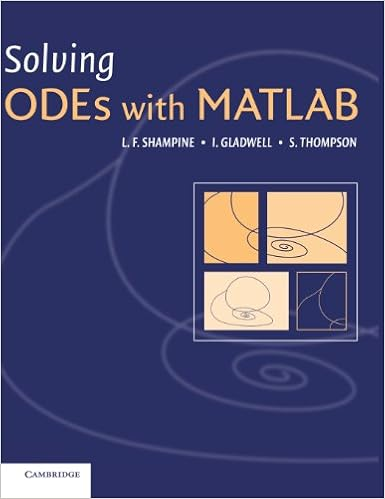离子通道一维泊松-能斯特-普朗克（Poisson-Nernst-Planck）模型- 阅读剩余部分 -

社会物理学- 阅读剩余部分 -

用Matlab bvp4c 解带未知参数的常微分方程边值问题，怎么给出合适的初值？\begin{equation*} \begin{split} f'''-R[(f')^2-ff'']+RA=&0\\ R''+Rfh'+1=&0\\ \theta ''+P_ef\theta '=&0 \end{split} \end{equation*}

\begin{equation*} \begin{split} f(0)=f'(0)=&0\\ f(1)=f'(1)=&1\\ h(0)=h(1)=&0\\ \theta(0)=&0\\ \theta(1)=&1 \end{split} \end{equation*}

- 阅读剩余部分 -

用Matlab bvp4c 解带未知参数的常微分方程边值问题\begin{equation*} y''+(100-\beta)y+\gamma y^3=0 \end{equation*}

\begin{equation*} \begin{split} y(-1)=&0\\ y(1)=&0\\ y(-1)=&0.1 \end{split} \end{equation*}

- 阅读剩余部分 -

动物集体行为背后，到底是何机制？